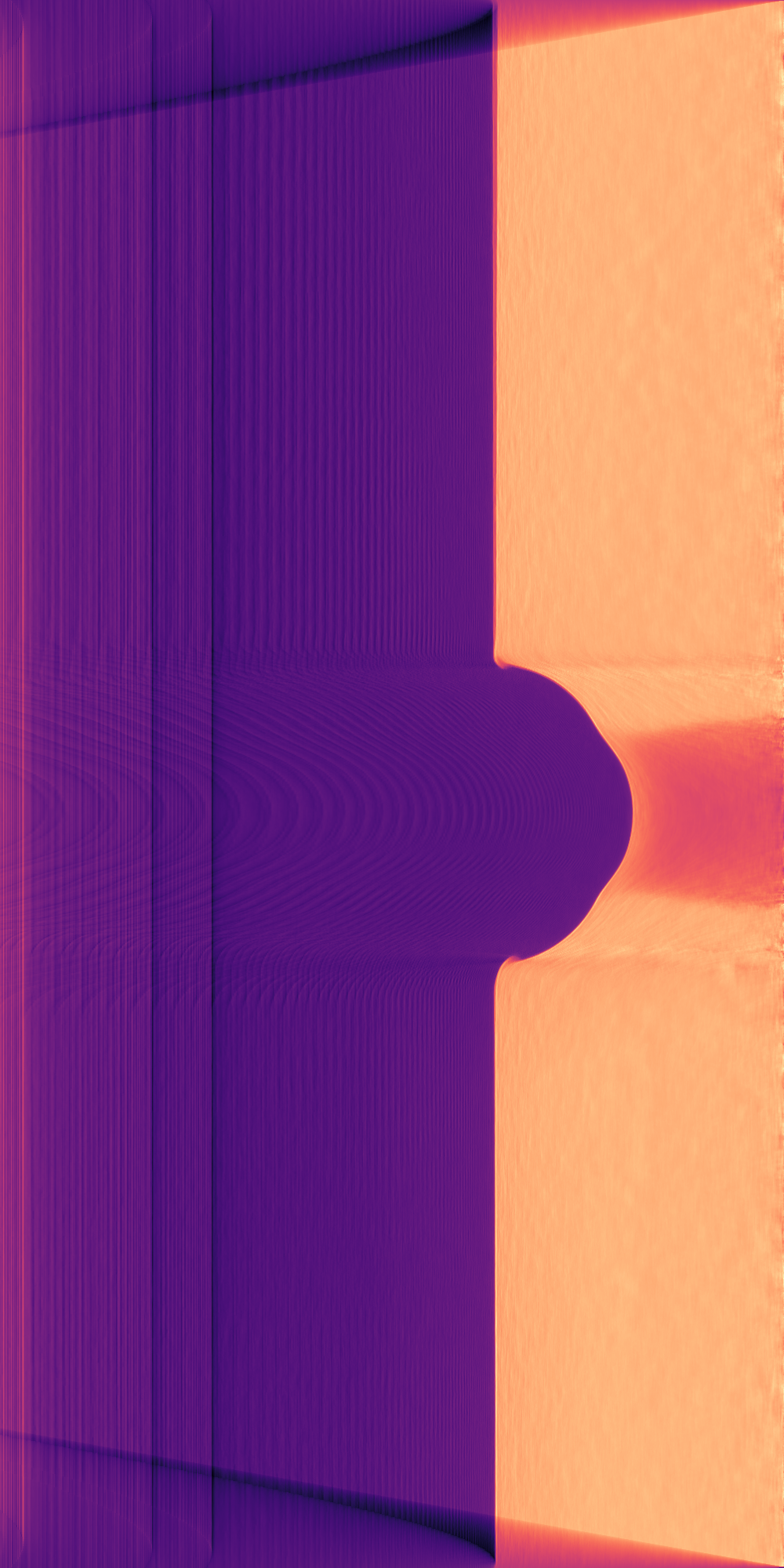# Quick start¶

Let’s now go through the necessary steps for doing reconstruction of a tomogram based on a single image. First, we need to import the tools:

import axitom as tom
from scipy.ndimage.filters import median_filter


The example data can be downloaded from the AXITOM/tests/example_data/ folder. The dataset was collected during tensile testing of a polymer specimen. Assuming that the example data from the repo is located in root folder, we can make a config object from the .xtekct file:

config = tom.config_from_xtekct("radiogram.xtekct")


We now import the projection:

projection = tom.read_image(r"radiogram.tif", flat_corrected=True)


As we will use a single projection only in this reconstruction, we will reduce the noise content of the projection by employing a median filter. This works fine since the density gradients within the specimen are relatively small. You may here choose any filter of your liking:

projection = median_filter(projection, size=21)


Now, the axis of rotation has to be determined. This is done be binarization of the image into object and background and determining the center of gravity of the object:

_, center_offset = tom.object_center_of_rotation(projection, background_internsity=0.9)


The config object has to be updated with the correct values:

config = config.with_param(center_of_rot=center_offset)


We are now ready to initiate the reconstruction:

tomo = tom.fdk(projection, config)


The results can then be visualized:

import matplotlib.pyplot as plt